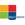# Activities

••• ##### Subject Area

• Math: Algebra I: Linear Functions
• Math: Algebra I: Quadratic Functions
• Math: Precalculus: Functions and Graphs

• ##### Author6-8
9-12

30 Minutes

• ##### Device
• TI-83 Plus Family
• TI-84 Plus
• TI-84 Plus Silver Edition
• ##### Other Materials
For more information on the Holt, Rinehart and Winston Mathematics Series, visit them on the web at www.hrw.com/math

## Explore Graphs and Factors

#### Activity Overview

Students use a graphing calculator to explore the relationship between the linear factors of a quadratic function and its zeros. They learn that the x-intercepts and axis of symmetry of a quadratic function can be determined from its linear factors.

This Technology Lab accompanies Lesson 5-3 from the ©2007 Holt, Rinehart and Winston Algebra 2 textbook.

#### Before the Activity

• Review the lesson PDF files.
• Make copies of the lesson PDF files for your class.

#### During the Activity

Students will:

• Graph linear and quadratic functions.
• Identify x-intercepts.
• Explore the relationship between the linear factors of a quadratic function and its zeros.
• Learn that the x-intercepts and axis of symmetry of a quadratic function can be determined from its linear factors.

#### After the Activity

Review student results:

• As a class, discuss questions that appeared to be more challenging
• Re-teach concepts as necessary